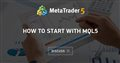# Second if statement is not executed after the first if statement's conditions comes false. My code is attached below. Please can someone point me in the right direction.

```//--- Create an instance of CTrade

input int NumberOfPositions = 3;
input double myLotSize = 0.20;

void OnTick()
{

double Bid = NormalizeDouble(SymbolInfoDouble(_Symbol, SYMBOL_BID),_Digits);
double Balance = AccountInfoDouble(ACCOUNT_BALANCE);
double Equity = AccountInfoDouble(ACCOUNT_EQUITY);

//---Get Price Data

MqlRates priceInformation[];

//---Sort it from current candles to older candle
ArraySetAsSeries(priceInformation, true);

//---Copy price data into array
int Data = CopyRates(Symbol(), Period(),0,Bars(Symbol(),Period()),priceInformation);

//--- RSI Strategy //---

//--- create array for the RSI
double myRSIArray[];

//---define RSI settings
int myRSIDefinition = iRSI(_Symbol, _Period,5,PRICE_CLOSE);

//--- sort price data from the current candle downwards
ArraySetAsSeries(myRSIArray, true);

//---Define EA, current candle, 3 candles, save in array
CopyBuffer(myRSIDefinition,0,0,3,myRSIArray);

//---calculate rsi value of the current candle
double myRSIValue = NormalizeDouble(myRSIArray,2);

//---Chart Output of the Current EA

Comment("myRsiValue: " , myRSIValue);

//--- check whether in uptrend or downtrend

//--- create an Array for several prices
double myMovingAverageArray[];

//--- define the properties of the Moving Average
int movingAverageDefinition = iMA(_Symbol,_Period,300,0,MODE_EMA,PRICE_CLOSE);

//---sort the price array from the current candle downwards
ArraySetAsSeries(myMovingAverageArray, true);

//--- Define EA, one line, current candle, 3 candles, store result
CopyBuffer(movingAverageDefinition,0,0,3,myMovingAverageArray);

//--- Calculate EA for the current candle
double myMovingAverageValue = myMovingAverageArray;

//---Chart output of the current EA
Comment("MovingAverageValue: ", myMovingAverageValue);

if ((priceInformation.close > myMovingAverageValue)&&(myRSIValue <= 30)&&
(PositionsTotal() < NumberOfPositions) && (Equity >= Balance))
{
myLotSize, //--- how much
NULL, //--- current symbol
(Bid-300000* _Point), //--- S.l
(Bid+1500000* _Point), //--- T.P
NULL //--- Comment
);

} else if ((priceInformation.close < myMovingAverageValue)&&(myRSIValue >= 70)&&
(PositionsTotal() < NumberOfPositions) && (Equity >= Balance))
{
//---sell
myLotSize, //--- how much
NULL, //--- current symbol
NULL //--- Comment
);

}
}```

Henihollar:

# Second if statement is not executed after the first if statement's conditions comes false. Please can someone point me in the right direction.

what makes you think that?   else if  says it does get executed. most likely the conditions of the second if are also false.

use the debugger and check the values

Henihollar: Second if statement is not executed after the first if statement's conditions comes false. Please can someone point me in the right direction.

Be careful of the if-else construct you are using. It can be very misleading.

This is what you are doing ...

```if ( condition1 )
{
// Something if ...
}
else if (condition2 )
{
// Something else if ...
};```

This is what it is actually doing ...

```if ( condition1 )
{
// Something if ...
}
else
{
if (condition2 )
{
// Something else if ...
};

// Something else ...
};```

You can write it like this for more control ...

```if ( condition1 )
{
// Something if ...
}
else
{
if (condition2 )
{
// Something else if ...
}
else
{
// Something else else ...
};

// Something else ...
};```

Henihollar :

You make a gross mistake: you create a new indicator handle on each tick. This is the biggest mistake!

According to the MQL5 style, the indicator handle is created ONCE!!! And this is done in OnInit.

Example:

### An example of get values from the iRSI indicator